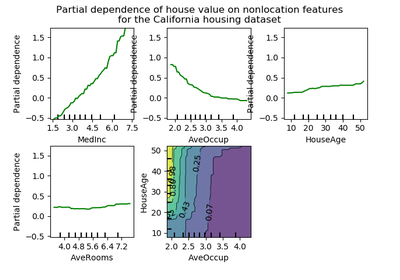# `sklearn.ensemble.partial_dependence`.plot_partial_dependence¶

`sklearn.ensemble.partial_dependence.``plot_partial_dependence`(gbrt, X, features, feature_names=None, label=None, n_cols=3, grid_resolution=100, percentiles=(0.05, 0.95), n_jobs=1, verbose=0, ax=None, line_kw=None, contour_kw=None, **fig_kw)[source]

Partial dependence plots for `features`.

The `len(features)` plots are arranged in a grid with `n_cols` columns. Two-way partial dependence plots are plotted as contour plots.

Read more in the User Guide.

Parameters: gbrt : BaseGradientBoosting A fitted gradient boosting model. X : array-like, shape=(n_samples, n_features) The data on which `gbrt` was trained. features : seq of ints, strings, or tuples of ints or strings If seq[i] is an int or a tuple with one int value, a one-way PDP is created; if seq[i] is a tuple of two ints, a two-way PDP is created. If feature_names is specified and seq[i] is an int, seq[i] must be < len(feature_names). If seq[i] is a string, feature_names must be specified, and seq[i] must be in feature_names. feature_names : seq of str Name of each feature; feature_names[i] holds the name of the feature with index i. label : object The class label for which the PDPs should be computed. Only if gbrt is a multi-class model. Must be in `gbrt.classes_`. n_cols : int The number of columns in the grid plot (default: 3). percentiles : (low, high), default=(0.05, 0.95) The lower and upper percentile used to create the extreme values for the PDP axes. grid_resolution : int, default=100 The number of equally spaced points on the axes. n_jobs : int The number of CPUs to use to compute the PDs. -1 means ‘all CPUs’. Defaults to 1. verbose : int Verbose output during PD computations. Defaults to 0. ax : Matplotlib axis object, default None An axis object onto which the plots will be drawn. line_kw : dict Dict with keywords passed to the `matplotlib.pyplot.plot` call. For one-way partial dependence plots. contour_kw : dict Dict with keywords passed to the `matplotlib.pyplot.plot` call. For two-way partial dependence plots. fig_kw : dict Dict with keywords passed to the figure() call. Note that all keywords not recognized above will be automatically included here. fig : figure The Matplotlib Figure object. axs : seq of Axis objects A seq of Axis objects, one for each subplot.

Examples

```>>> from sklearn.datasets import make_friedman1
## Examples using `sklearn.ensemble.partial_dependence.plot_partial_dependence`¶